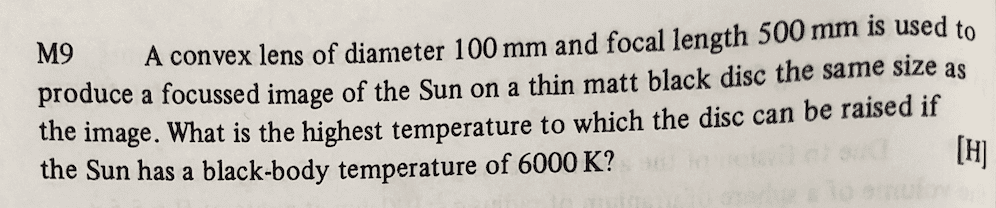# Problem on thermal radiation and lenses

phantomvommand
Homework Statement:
Relevant Equations:
P (rad) = sigma e0 T^4
At long distances, images due to convex lenses are formed at the foci.I know that the Sun is at a very far distance from the lens, so I assume the rays are parallel and the image is formed at the focus (500mm away from the centre of the lens).
How do I calculate the diameter of the image?
The hint to solving this problem says that "The angular diameters of object and image are equal". What is the "angular diameter", and what does the hint mean? If the light rays intersect at a focal point, how can there even be a diameter? Furthermore, how is the fact that the lens diameter = 100mm useful?

I am quite confused by this question, any help is appreciated. Thank you!

Homework Helper
Gold Member
2022 Award
Homework Statement:: Please see the attached photo
Relevant Equations:: P (rad) = sigma e0 T^4
At long distances, images due to convex lenses are formed at the foci.

View attachment 279679
I know that the Sun is at a very far distance from the lens, so I assume the rays are parallel and the image is formed at the focus (500mm away from the centre of the lens).
How do I calculate the diameter of the image?
The hint to solving this problem says that "The angular diameters of object and image are equal". What is the "angular diameter", and what does the hint mean? If the light rays intersect at a focal point, how can there even be a diameter? Furthermore, how is the fact that the lens diameter = 100mm useful?

I am quite confused by this question, any help is appreciated. Thank you!
A ray arriving from a point at one edge of the sun, as observed from Earth, is not quite parallel with a ray from the opposite edge, so they will not focus at the same point.
OTOH, rays from one point on the sun arriving at different points of the lens are effectively parallel, so do focus at the same point.
As a result, an actual image of the sun is produced, spots and all.

phantomvommand
A ray arriving from a point at one edge of the sun, as observed from Earth, is not quite parallel with a ray from the opposite edge, so they will not focus at the same point.
OTOH, rays from one point on the sun arriving at different points of the lens are effectively parallel, so do focus at the same point.
As a result, an actual image of the sun is produced, spots and all.
•